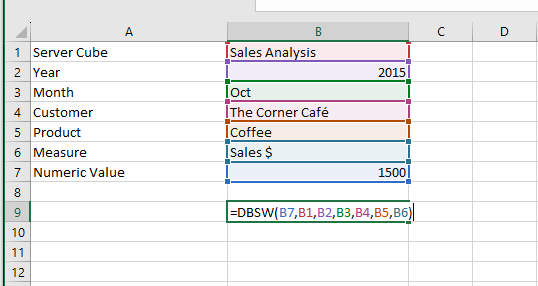# DBSW TM1 Excel Syntax and Use

The DBSW function in TM1 sends a numeric value to a TM1 cube.

## Syntax

The syntax for using DBSW function is:

``DBSW(value, cube, e1, e2[,...en])``

Where:

• Value: The numeric value that is being sent to the cube.
• Cube: The specific cube in TM1 to which the value is being sent.
• e1, …en: These represent the names of elements that define the intersection within the cube where the value is being sent.

The arguments e1 through en are sequence sensitive, meaning the order of the elements is important. e1 corresponds to an element from the first dimension of the cube, e2 corresponds to an element from the second dimension, and so on. It’s worth noting that these arguments can also be the names of aliases for dimension elements if applicable.

Also, if any of the element names are numeric, they must be enclosed in quotation marks when used as arguments in the DBSW function.

## Example of DBSW

We have a Sales Analysis cube with the following dimensions: Year, Month, Customer, Product, Measures. Suppose we want to send a numeric value of 1500 to the intersection of the following elements in the “Sales Analysis” cube:

• Year: 2015
• Month: Oct
• Customer: The Corner Cafe
• Product: Coffee
• Measure: Sales \$

## Usage

Continuing the example from above, we can use the following formula in Excel:

``DBSW(1500, "Sales Analysis", "2015", "Oct", "The Corner Cafe", "Coffee", "Sales \$")``

In this example, the value 1500 is being sent to the specified intersection within the “Sales Analysis” cube. The Year is 2015, Month is Oct, Customer is The Corner Cafe, Product is Coffee, and Measure is Sales \$.Remember, in Planning Analytics for Excel (PAX), we like to keep things flexible and dynamic! Instead of sticking to old hard-coded values, we prefer referencing values from other cells. This approach lets us easily tweak and update the values in our reports.

## Some Important Things…

### Difference between DBSW and DBS

In worksheets with numerous cube references, the DBSW function optimizes value transmission by grouping them together instead of sending them individually. Unlike regular DBS functions that are updated individually during recalculation, DBSW references enable TM1 to send all modified values within a worksheet in a single bundle.

In situations where this applies, you can confidently use a DBS or DBR function as an argument within a DBS function.

### No Strings (Attached)

This function can’t be used for sending strings to a TM1 cube. To do this, please use a DBSS instead.

### Visual Basic Calculations

Please note that if you use VBA to calculate a worksheet containing DBSW functions, it is necessary to invoke the TM1 macro function for worksheet calculation. Avoid using the VB Calculate method as it executes each DBSW function individually, negating the intended efficiency and resulting in decreased performance.

### Excel Only

Lastly, it’s important to emphasise that this worksheet function is only valid within Excel worksheets.